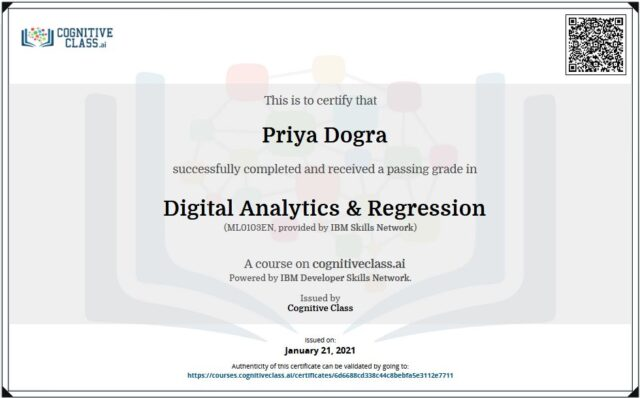Home Cognitive Class Digital Analytics & Regression Cognitive Class Answers

# Digital Analytics & Regression Cognitive Class Answers

0## Enroll Here: Digital Analytics & Regression Certificate IBM – Cognitive Class

Module 1: Case Study ‘CEO vs. CMO’

Question 1: You are a newly-hired analyst at a small tech startup in a big metropolitan city. In your first team meeting, and one week before the scheduled product launch, the Chief Executive Officer (CEO) and the Chief Marketing Officer (CMO) have a heated argument about what to call the product.

The CEO has done a quick search on Google Trends and found that ‘Analytics’ is, by far, a more popular search term than ‘Data Science’ or ‘Data Scientist’. The CMO, who has some experience in SEM from his work at another tech company, has a gut feeling that ‘Data Scientist Workbench’ will bring the right target group to the new product. The two executives ask you to weigh in.

Based on the Case Study you have read, which of the following is the most suitable data set to start an analysis to help the executives decide which term to include in the product name?

• Open Data
• Search Engine Trends Data
• Survey Data

Question 2: Based on the Case Study you have read, how many domains available for purchase?

• 1
• 2
• 3
• 4

Question 3: Based on the Case Study you have read, what was the ‘Expert Tip’?

• Don’t offer your opinion to senior executives if you are new to a company.
• Your first assumption is always the correct one.
• If it can’t be measured, it doesn’t exist.
• When solving business problems with data, be curious.

Module 2: Importing Google Trends data in R

Question 1: How many rows does the function head(dataset) return?

Question 2: What does head(case_table\$WeekID) do?

• returns the first six rows of case_table and all of its columns
• returns all the data in case_table
• returns the first six items of the WeekID column in case_table
• returns the entire WeekID column of data in case_table

Question 3: TRUE or FALSE? The function str() can be used to tell you the number of rows and columns in a dataframe, and some other characteristics of the data.

• True
• False

Module 3: Plotting & Correlation

Question 1: Which search term has the most number of searches?

• Machine Learning
• Data Scientist
• Analytics
• Data Science
• Regression

Question 2: A correlation score of 0.9 between variables X and Y indicate which of the following?

• As X increases, Y decreases.
• There is a weak positive correlation between X and Y.
• X and Y are opposites of each other.
• A strong positive correlation.
• X causes Y.

Question 3: In the R script file, which command can you use to plot line graphs?

• makePlot( )
• createGraph( )
• plot( )
• linegraph( )
• graph( )

Module 4: Simple Linear Regression in R

Question 1: What is the correlation between searches for ‘data science’ and ‘data scientist’? Give the numeric answer to two decimal places.

Question 2: Running a linear model in R for a dataset results in the formula: Y = 5.0 + 3.0X. Which of the following is true?

• Y will always be positive.
• X is 3 times as large as Y.
• There is a negative correlation between X and Y.
• As X increases, Y decreases.
• When X is equal to 5, the model predicts that Y will equal 20.

Question 3: In R, what symbol or character do we use to specify a column in a table?

• ?
• ==
• /
• \$

Digital Analytics & Regression Final Exam Answers – Cognitive Class

Question 1: What is the correlation (as a %) between the two search terms in the exam data set? For example, if the correlation is 0.5, enter 50 below.

Question 2: What is the mean of the values for Hadoop searches? Your answer should include two decimal places (for example, 10.01).

Question 3: What is the R Squared for the Regression model in the exam data set? The answer format must be a percentage with no decimal places. For example, if the R^2 value is 0.5, then you should write 50 below.

Question 4: What weeks correspond to WeekID 16?

• 2004-06-06 – 2004-06-12
• 2004-01-25 – 2004-01-31
• 2004-04-18 – 2004-04-24
• 2004-08-15 – 2004-08-21

Question 5: Which R function creates a linear model?

• lm ( )
• abline ( )
• regression ( )
• summary ( )

Question 6: Determine the formula for your linear model. Using the linear model you have just created to predict search index values: if the search index for ‘Hadoop’ is 35, what is the predicted corresponding search index for ‘Big Data’ according to your model?

• 88
• 19
• 45
• 30

Question 7: Generally speaking, the simple linear model created from these two search terms fits the data well, TRUE/FALSE?

• TRUE
• FALSE

Question 8: In the Linear Model, ‘Hadoop’ is the Y variable, TRUE/FALSE?

• TRUE
• FALSE

Question 9: Having now gone through the exercises in this course, and going back to the debate between the CEO vs. CMO, what product name was chosen as the most suitable for the target audience?

• Analytics Workbench
• Data Scientist Workbench